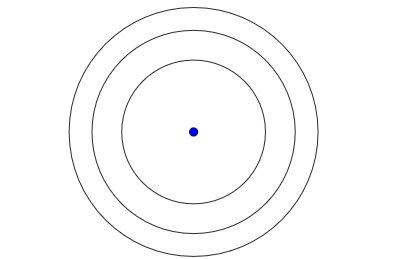# 3 Circles

Geometry Level 3

Three circles have the same centre. The radius of the smaller circle is $\sqrt { 3 }$ The area of the region between the larger circle and the one in the middle is equal to the area of the smaller circle, and the area between the one in the middle and the smaller is equal to the area of the smaller circle. What is the radius of the larger circle?×